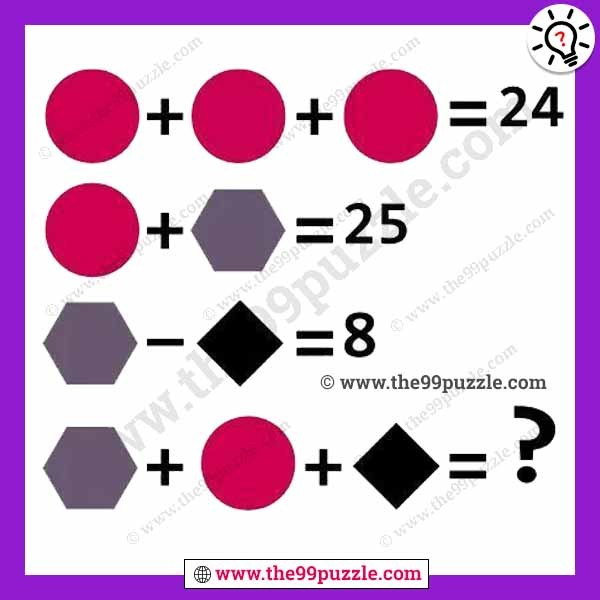# Shape picture math equation with answer – Puzz132

In this shape, picture math equation is very important in many entrance tests. Simple math picture puzzles can improve your logical thinking. If you are a genius you can solve the shape picture math equation. Find out the value of the last equation?Circle = 8

Hexagon=17

Square=9

8+8+8=24

8+17=25

17−9=8

17+8+9=34